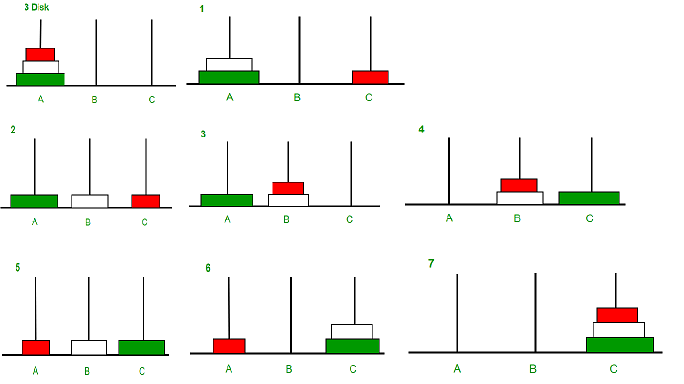Open in App
Not now

# C Program for Tower of Hanoi

• Difficulty Level : Easy
• Last Updated : 20 Dec, 2022

Tower of Hanoi is a mathematical puzzle where we have three rods and n disks. The objective of the puzzle is to move the entire stack to another rod, obeying the following simple rules: 1) Only one disk can be moved at a time. 2) Each move consists of taking the upper disk from one of the stacks and placing it on top of another stack i.e. a disk can only be moved if it is the uppermost disk on a stack. 3) No disk may be placed on top of a smaller disk.## C

 `#include ` `// C recursive function to solve tower of hanoi puzzle``void` `towerOfHanoi(``int` `n, ``char` `from_rod, ``char` `to_rod, ``char` `aux_rod)``{``    ``if` `(n == 1)``    ``{``        ``printf``(``"\n Move disk 1 from rod %c to rod %c"``, from_rod, to_rod);``        ``return``;``    ``}``    ``towerOfHanoi(n-1, from_rod, aux_rod, to_rod);``    ``printf``(``"\n Move disk %d from rod %c to rod %c"``, n, from_rod, to_rod);``    ``towerOfHanoi(n-1, aux_rod, to_rod, from_rod);``}` `int` `main()``{``    ``int` `n = 4; ``// Number of disks``    ``towerOfHanoi(n, ``'A'``, ``'C'``, ``'B'``);  ``// A, B and C are names of rods``    ``return` `0;``}`

Output:

``` Move disk 1 from rod A to rod B
Move disk 2 from rod A to rod C
Move disk 1 from rod B to rod C
Move disk 3 from rod A to rod B
Move disk 1 from rod C to rod A
Move disk 2 from rod C to rod B
Move disk 1 from rod A to rod B
Move disk 4 from rod A to rod C
Move disk 1 from rod B to rod C
Move disk 2 from rod B to rod A
Move disk 1 from rod C to rod A
Move disk 3 from rod B to rod C
Move disk 1 from rod A to rod B
Move disk 2 from rod A to rod C
Move disk 1 from rod B to rod C```

Time Complexity: O(2n)

Auxiliary Space: O(n)

Please refer complete article on Program for Tower of Hanoi for more details!

My Personal Notes arrow_drop_up